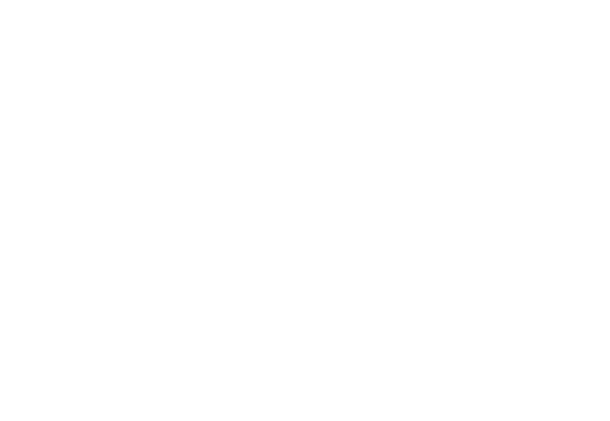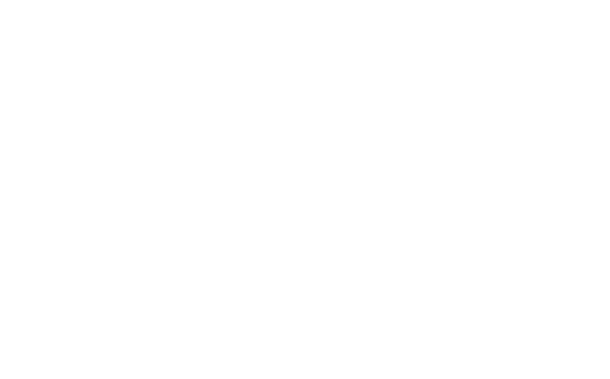# How Many Triangles Can You See In This Picture? The Answer Has Divided The Internet

This geometry problem has everyone in a fix! This new brain teaser is a mathematical puzzle which asks users to calculate the number of triangles in the picture, and everyone is left scratching their head!

This drawing was created by Kumar Ankit on quora.com and since then, it has gone viral after being shared hundreds of thousands of times on Facebook.The sketch shows a segmented triangle and asks people to calculate the number of triangles in it.

The answers have been erratic, ranging from 4 to even 44! Can you figure out the correct answer yourself? Just give it a try!The correct answer is actually 25; 24 triangles contained within the main triangle and a sneaky triangle hidden in the artist’s signature.

Still can’t get the answer? You can work it out as well if you know the strategy!

There are six triangles in the top row – three individuals, two pairs, and one triangle made up of all three.

Similarly, there are six in the second row, six in the third row, and six in the fourth row.

This makes 24, with a hidden triangle in the signature of the artist.

Martin Silvertant, a mathematician, explains the strategy using this simple diagram.Want another brain teaser? Check this one out.

http://wonderfulengineering.com/people-couldnt-solve-math-test/

Did you get the right answer? Let us know in the comments’ section below!